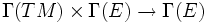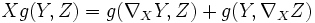# Metric connection

This lives as an element of: the space of all connections, which in turn sits inside the space of all$\R$-bilinear maps$\Gamma(TM) \times \Gamma(E) \to \Gamma(E)$

## Definition

### Given data

A differential manifold$M$.

A metric bundle$E$ over$M$ (viz, a vector bundle with a smoothly varying metric structure$g$ on each fibre of$E$ over$M$).

### Definition part

A metric connection on$(M,g)$ is a connection$\nabla$ on the vector bundle$E$ over$M$ satisfying the following condition:$X g (Y,Z) = g (\nabla_X Y, Z) + g(Y, \nabla_X Z)$

Here$X$ is a vector field (viz a section of$TM$) and$Y$ and$Z$ are sections of$E$.

In other words, it is a connection such that the dual connection on the dual bundle to$E$ is the same as the connection obtained by the natural isomorphism between$E$ and its dual (induced by the metric).

We are in particular interested in metric linear connections, which are metric connections over the tangent bundle for a Riemannian manifold (viz, the tangent bundle is endowed with a Riemannian metric). Of these, a very special one is the Levi-Civita connection, which is the only torsion-free metric linear connection.